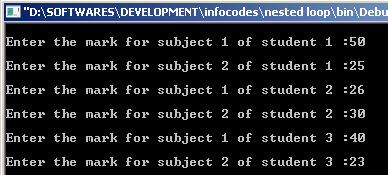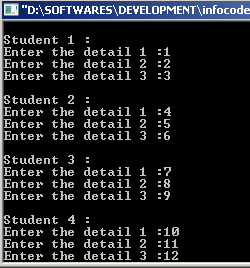# Nested Loops in C Programming

Program Abstraction:    This tutorial explains the basic concepts of Nested Loops loops in C with examples.
We already leant about nested if-else statement in a previous lesson. Where, an ‘if statement’ contains another ‘if statement’. Like this, in nested loop, one loop contains another loop. It may be any type of loop, such as for, while and do –while.
Where we use nested loops?

Let us learn with an example: There are 3 students and a program which ask their marks in two subjects one by one. At first, student 1 was selected, and his marks in both subjects were accepted, and then second student elected and his marks were accepted and so on. Here first loop (variable i) select students one by one and entering marks in both subjects is by second loop. First loop has duty of selecting students and second loop has duty of accepting marks.Here is an example code which accepts 2 marks of 3 stuents:

 #include #include void main() {     int i;     int j;     int mark;     for(i = 1; i<=3;i++)         for(j =1;j<=2;j++)     {         printf(“n Enter the mark for subject %d of student %d :”, j , I );         scanf(“%d”, &mark);     } }

Output:How the codes work?

First ‘for loop’ controls the number of students with the variable i, which has 3 iteration; 1 to 3. Second loop controls marks of two subjects. First the value of i become 1, that means the student 1 was elected and entered into second loop, where the value of j is 1, means his marks in subject 1 will be accepted. Then value of j incremented and mark in subject 2 was accepted. After completing iteration in loop second, then program control goes into first loop, then the value of i become incremented; means the student 2 selected. Again go to second loop then accept marks in both subjects using incrementing value of j. And so on.
In above example, variable ‘mark’ is a dummy, all marks in all students saved in same variable. Not for implementation, only for study purpose.
Note that, if the first loop takes a value and entered into second loop, then the program control return into first loop after executing all the iterations in second loop, means next increment of first loop will be after the completion of second loop iteration. After incrementing first loop, again entered into second loop, that will completer all iteration. In above example, first loop will work at 1 times completely, but second loop will execute 3 times, because first loop has 3 iteration.

Example 2: For accepting 10 students’ details. (Note that the accepting variable is a dummy integer)

 #include #include void main() {     int i;     int j;     int mark;     for(i = 1; i<=10;i++)        {               printf(“nStudent %d :n”,i);                for(j =1;j<=3;j++)               {                  printf(“Enter the detail %d :”,j);                  scanf(“%d”,&mark);                }         } }

Here first student is elected in first loop, and in nested loop his 3 details will be collected. if the first loop contains any extra statement than second loop, then should use an additional braze  {  }.
Output: